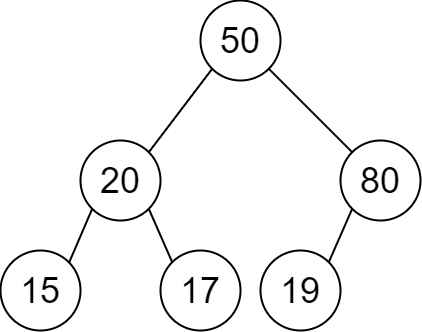# 2196. Create Binary Tree From Descriptions

Medium
You are given a 2D integer array `descriptions` where `descriptions[i] = [parenti, childi, isLefti]` indicates that `parenti` is the parent of `childi` in a binary tree of unique values. Furthermore,
• If `isLefti == 1`, then `childi` is the left child of `parenti`.
• If `isLefti == 0`, then `childi` is the right child of `parenti`.
Construct the binary tree described by `descriptions` and return its root.
The test cases will be generated such that the binary tree is valid.
Example 1:Input: descriptions = [[20,15,1],[20,17,0],[50,20,1],[50,80,0],[80,19,1]]
Output: [50,20,80,15,17,19]
Explanation: The root node is the node with value 50 since it has no parent.
The resulting binary tree is shown in the diagram.
Example 2:Input: descriptions = [[1,2,1],[2,3,0],[3,4,1]]
Output: [1,2,null,null,3,4]
Explanation: The root node is the node with value 1 since it has no parent.
The resulting binary tree is shown in the diagram.
Constraints:
• `1 <= descriptions.length <= 10^4`
• `descriptions[i].length == 3`
• `1 <= parenti, childi <= 10^5`
• `0 <= isLefti <= 1`
• The binary tree described by `descriptions` is valid.

### 解題

/**
* Definition for a binary tree node.
* type TreeNode struct {
* Val int
* Left *TreeNode
* Right *TreeNode
* }
*/
func createBinaryTree(descriptions [][]int) *TreeNode {
m := make(map[int]*TreeNode) // record *TreeNode
children := make(map[int]bool) // record children
for _, des := range descriptions { // add children to each TreeNode
if _, ok := m[des]; !ok {
m[des] = &TreeNode{ Val: des }
}
if _, ok := m[des]; !ok {
m[des] = &TreeNode{ Val: des }
}
if des == 1 {
m[des].Left = m[des]
} else {
m[des].Right = m[des]
}
children[des] = true
}
// head : never be a child
for k := range m {
if _, ok := children[k]; !ok {
return m[k]
}
}
return nil
}